# Wilcox Solar Observatory Magnetograms

## #8250 - 8259, 2005.04.18 - 2005.05.01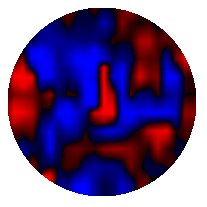```WSO Magnetogram sn=8250	 observed at 2005:04:18_22h:24m:03s
Central Meridian Carrington Longitude CT2028:032
Sky = 0 (5 worst)
trouble=00004000 NOTE:
12 intensity variance exceeds 5.0
```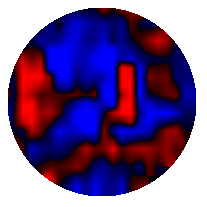```WSO Magnetogram sn=8251	 observed at 2005:04:19_22h:02m:34s
Central Meridian Carrington Longitude CT2028:019
Sky = 0 (5 worst)
trouble=00004000 NOTE:
12 intensity variance exceeds 5.0
``````WSO Magnetogram sn=8252	 observed at 2005:04:20_19h:36m:32s
Central Meridian Carrington Longitude CT2028:007
Sky = 0 (5 worst)
trouble=00004000 NOTE:
12 intensity variance exceeds 5.0
```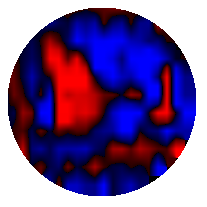```WSO Magnetogram sn=8253	 observed at 2005:04:21_22h:52m:03s
Central Meridian Carrington Longitude CT2029:352
Sky = 1 (5 worst)
trouble=00004000 NOTE:
12 intensity variance exceeds 5.0
```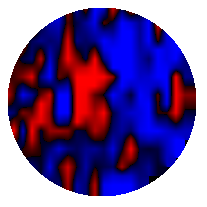```WSO Magnetogram sn=8254	 observed at 2005:04:22_19h:38m:11s
Central Meridian Carrington Longitude CT2029:340
Sky = 3 (5 worst)
trouble=00004000 NOTE:
12 intensity variance exceeds 5.0
```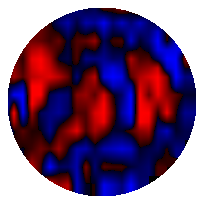```WSO Magnetogram sn=8255	 observed at 2005:04:25_19h:04m:18s
Central Meridian Carrington Longitude CT2029:301
Sky = 3 (5 worst)
trouble=00004000 NOTE:
12 intensity variance exceeds 5.0
```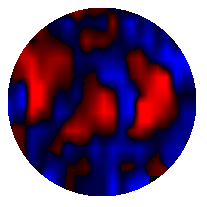```WSO Magnetogram sn=8256	 observed at 2005:04:25_21h:33m:03s
Central Meridian Carrington Longitude CT2029:300
Sky = 0 (5 worst)
trouble=00004000 NOTE:
12 intensity variance exceeds 5.0
```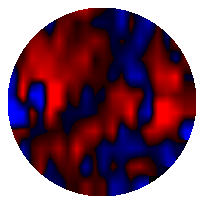```WSO Magnetogram sn=8257	 observed at 2005:04:26_22h:08m:24s
Central Meridian Carrington Longitude CT2029:286
Sky = 1 (5 worst)
trouble=00004000 NOTE:
12 intensity variance exceeds 5.0
```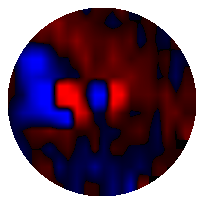```WSO Magnetogram sn=8258	 observed at 2005:04:29_17h:24m:21s
Central Meridian Carrington Longitude CT2029:249
Sky = 0 (5 worst)
trouble=00004000 NOTE:
12 intensity variance exceeds 5.0
```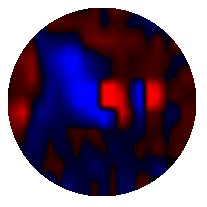```WSO Magnetogram sn=8259	 observed at 2005:05:01_16h:32m:06s
Central Meridian Carrington Longitude CT2029:223
Sky = 2 (5 worst)
trouble=00004000 NOTE:
12 intensity variance exceeds 5.0
```